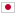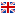Contest Duration: ~ (local time) (300 minutes) Back to Home
F - Endless BFS/Time Limit: 2 sec / Memory Limit: 512 MB

### Problem Statement

Mr. Endo wanted to write the code that performs breadth-first search (BFS), which is a search algorithm to explore all vertices on an undirected graph. An example of pseudo code of BFS is as follows:

1: $current \leftarrow \{start\_vertex\}$ 2: $visited \leftarrow current$ 3: while $visited \ne$ the set of all the vertices 4: $found \leftarrow \{\}$ 5: for $v$ in $current$ 6: for each $u$ adjacent to $v$ 7: $found \leftarrow found \cup \{u\}$ 8: $current \leftarrow found \setminus visited$ 9: $visited \leftarrow visited \cup found$

However, Mr. Endo apparently forgot to manage visited vertices in his code. More precisely, he wrote the following code:

1: $current \leftarrow \{start\_vertex\}$ 2: while $current \ne$ the set of all the vertices 3: $found \leftarrow \{\}$ 4: for $v$ in $current$ 5: for each $u$ adjacent to $v$ 6: $found \leftarrow found \cup \{u\}$ 7: $current \leftarrow found$

You may notice that for some graphs, Mr. Endo's program will not stop because it keeps running infinitely. Notice that it does not necessarily mean the program cannot explore all the vertices within finite steps. See example 2 below for more details.Your task here is to make a program that determines whether Mr. Endo's program will stop within finite steps for a given graph in order to point out the bug to him. Also, calculate the minimum number of loop iterations required for the program to stop if it is finite.

### Input

The input consists of a single test case formatted as follows.

$N$ $M$ $U_{1}$ $V_{1}$ $\vdots$ $U_{M}$ $V_{M}$

The first line consists of two integers $N$ ($2 \le N \le 100{,}000$) and $M$ ($1 \le M \le 100{,}000$), where $N$ is the number of vertices and $M$ is the number of edges in a given undirected graph, respectively. The $i$-th line of the following $M$ lines consists of two integers $U_{i}$ and $V_{i}$ ($1 \le U_{i}, V_{i} \le N$), which means the vertices $U_{i}$ and $V_{i}$ are adjacent in the given graph. The vertex 1 is the start vertex, i.e. $start\_vertex$ in the pseudo codes. You can assume that the given graph also meets the following conditions.

• The graph has no self-loop, i.e., $U_{i} \ne V_{i}$ for all $1 \le i \le M$.
• The graph has no multi-edge, i.e., $\{U_{i}, V_{i}\} \ne \{U_{j}, V_{j}\}$ for all $1 \le i < j \le M$.
• The graph is connected, i.e., there is at least one path from $U$ to $V$ (and vice versa) for all vertices $1 \le U, V \le N$.

### Output

If Mr. Endo's wrong BFS code cannot stop within finite steps for the given input graph, print -1 in a line. Otherwise, print the minimum number of loop iterations required to stop.

### Sample Input 1

3 3
1 2
1 3
2 3


### Output for Sample Input 1

2


### Sample Input 2

4 3
1 2
2 3
3 4


### Output for Sample Input 2

-1


Transition of $current$ is $\{1\} \rightarrow \{2\} \rightarrow \{1, 3\} \rightarrow \{2, 4\} \rightarrow \{1, 3\} \rightarrow \{2, 4\} \rightarrow \cdots$. Although Mr. Endo's program will achieve to visit all the vertices (in 3 steps), $current$ will never become the same set as all the vertices.

### Sample Input 3

4 4
1 2
2 3
3 4
4 1


### Output for Sample Input 3

-1


### Sample Input 4

8 9
2 1
3 5
1 6
2 5
3 1
8 4
2 7
7 1
7 4


### Output for Sample Input 4

3